MORE IN Circuits and Transmission Lines
MU Electronics and Telecom Engineering (Semester 3)
Circuits and Transmission Lines
May 2013
Total marks: --
Total time: --
INSTRUCTIONS
(1) Assume appropriate data and state your reasons
(2) Marks are given to the right of every question
(3) Draw neat diagrams wherever necessary

1 (a) Determine K for the given network.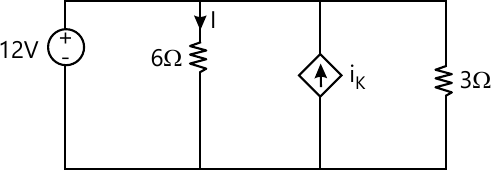4 M
1 (b) The reduced incidence matrix of an oriented graph is -
$A=\begin{bmatrix} 0 &-1 &1 &0 &0 \\ 0&0 &-1 &-1 &-1 \\ -1&0 &0 &0 &1 \end{bmatrix}$ Draw oriented graph and how many trees are possible for this graph.
4 M
1 (c) For the circuit shown, Vc is 0 at t= 0 sec. Find Icctj for t > 0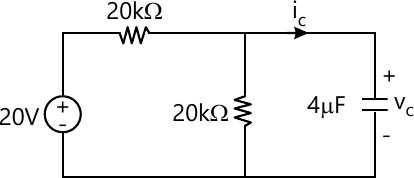4 M
1 (d) For the circuit shown below, find current I: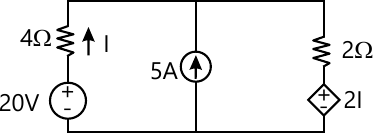4 M
1 (e) Derive the expression for transmission parameters in terms of Z parameters.
4 M

2 (a) Linear graph of a network is shown below. For the given tree (shown with firm lines) obtain -
(i) Fundamental cutset matrix
(ii) Fundamental tieset matrix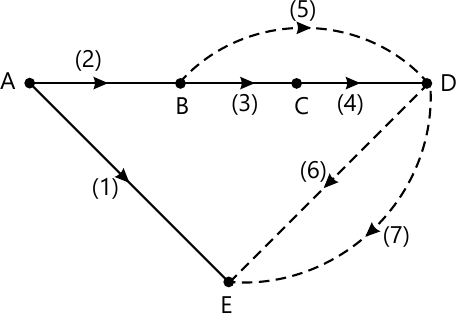10 M
2 (b) A series R-L circuit has a constant voltage 25V applied at t = 0. At what time does VR = VL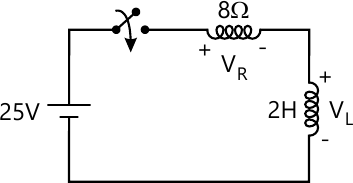10 M

3 (a) Find the voltage across 6Ω resistor using mesh analysis.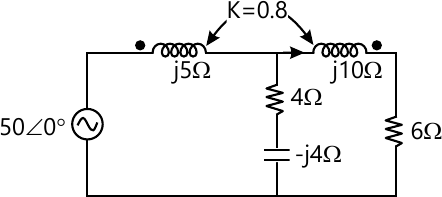10 M
3 (b) In the given circuit, switch is charged from position 1 to position 2 at t= 0, steady state has been reached before switching. Find the values of
$i, \dfrac{di}{dt} \ and \ \dfrac{d^2i}{dt^2} at t=0^+$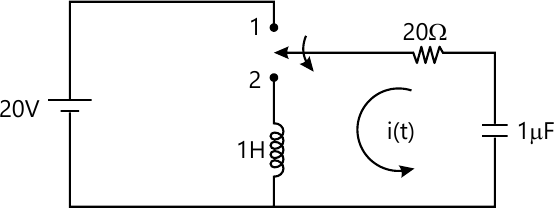10 M

4 (a) Find the current through 50Ω resistor using Thevenin's theorem.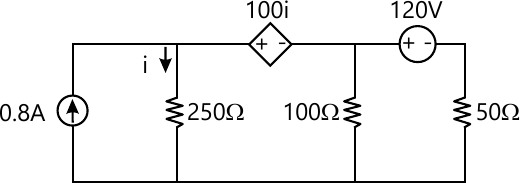10 M
4 (b) Find the h-parameter for the network shown below.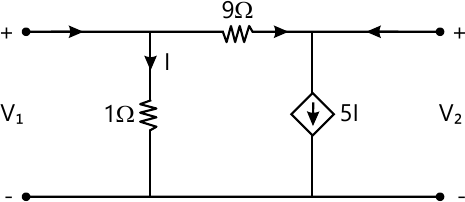10 M

5 (a) Determine whether following functions are positive real. $(i)\ \dfrac {s(s+3)(s+5)}{(s+1)(s+4)} \\ (ii)\ \dfrac {2s^2+2s+4}{(s+1)(s^2+2)}$
10 M
5 (b) Check whether following polynomials are Hurwitz or not
$(i) s^3+4s^2+5s+2 \\ (ii) s^4+s^3+2s^2+3s+2$
4 M
5 (c) For the network shown below, find $\dfrac {V_C}{Y}$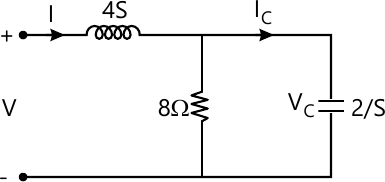6 M

6 (a) Realise the following function in Cauer-I and Cauer-II from
$Z(s)=\dfrac {(s+1)(s+3)}{(s+2)(s+6)}$
10 M
6 (b) Find Z parameter for the network shown below.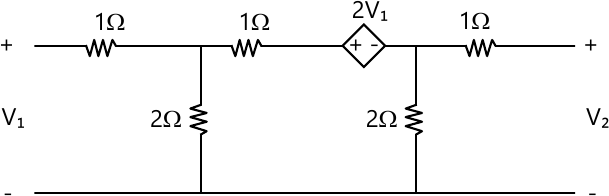10 M

7 (a) Find V2/V1 and I2/I1 for the network shown below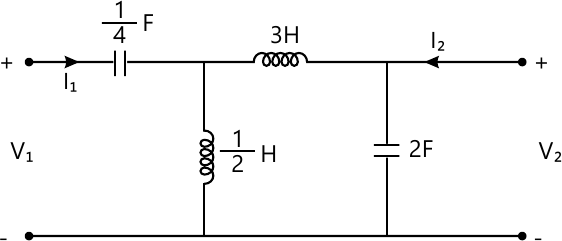10 M
7 (b) For the network shown below reaches steady with switch K opened. At t=0, the switch is closed, find i(t) for t> 0.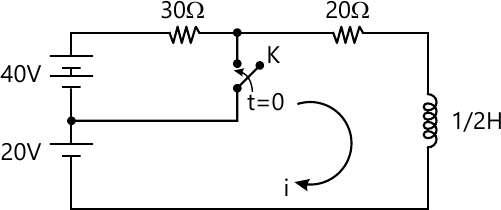10 M

More question papers from Circuits and Transmission Lines